## 7.15Summary of turbulence modelling

• The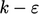model solves transport equations for turbulent kinetic energy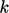and dissipation rate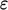, Sec. 7.1 .
• It is the original of a family of two-equation models, which ultimately provide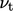to calculate the turbulent stresses.
• Initial and inlet values for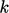andmust be speciﬁed, which can be calculated from turbulent intensity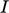and mixing length scale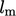, respectively, Sec. 7.2 .
•andare often estimated using functions that ﬁt experimental data for fully developed turbulent ﬂow, Sec. 7.3 .
• The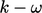models replaceby speciﬁc dissipation rate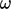, with equivalent expressions for initial and inlet values, Sec. 7.10 .
• The two “standard”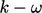models today are the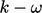SST model and2006 model, Sec. 7.11 .
• Models can provide a turbulent conductivity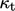to calculate the turbulent heat ﬂux for thermal problems, Sec. 7.12 .

### Turbulent boundary layers

• Turbulent boundary layers include a thin viscous sub-layer adjacent to the boundary with a linear velocity proﬁle and, further from the boundary, the inertial sub-layer with a log law proﬁle, Sec. 7.4 .
• Proﬁles in temperature are similar to those for velocity, with equivalent linear and log law relationships, Sec. 7.13 .
• Very thin cells are generally needed to resolve the viscous sub-layer to calculate the velocity gradient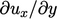at the wall accurately, Sec. 7.5 .
• Such thin cells within the boundary layer region can increase the mesh to a size which is prohibitively costly to run.

### Wall functions

• Wall functions permit much larger cells near the wall, by exploiting the universal character of the velocity distribution.
• The functions increase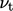at the wall to compensate for the under-prediction ofwith larger cells, to improve the prediction of the wall shear stress, Sec. 7.5 .
• Thermal wall functions similarly increase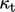at the wall to compensate for the under-prediction of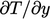, in order to improve the prediction of the wall heat ﬂux, Sec. 7.14 .
• Standard wall functions make no adjustment towhen the near wall cell centre falls below the transition within a buﬀer layer, Sec. 7.5 .
• Other models include a continuous function ofthrough the viscous sub-layer to the wall and adjustments for surface roughness, Sec. 7.6 .
• Boundary conditions for turbulence ﬁelds with wall functions are based on observed proﬁles of those ﬁelds, Sec. 7.7 .

### Models with resolved boundary layers

• Turbulence models must predict the universal character of boundary layers when the viscous sub-layer is resolved with suﬃciently thin cells, Sec. 7.8 .
• Models like the Launder-Sharma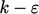include source terms and damping functions to improve the predictions and to simplify boundary conditions, Sec. 7.9 .
Notes on CFD: General Principles - 7.15 Summary of turbulence modelling